# Small Tricks

Here's a few, unrelated things that may be cool or helpful.

## Multiplying two complicated numbers

Let's say you're trying to multiply 78 and 44 together. Theres a lot of tricks and structures you can use to help you, but I'm going to show you mine.

Create a row of numbers, all of which add up to one of your operands, and then create a column of numbers, all of which add up to your other operand. Intersect them at the top left corner using the number 1 as the corner number. It's helpful to separate out the numbers so that only one non-zero digit exists in each number. See below.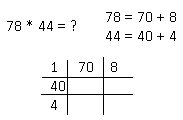The idea is you're going to make a grid, and for each blank cell, multiply the two numbers which share that row and column. Do this for all empty cells, then sum up the results of all those values and you'll end up with the solution to your original problem!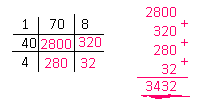One neat thing about this method is that you can tune the difficulty to your ability to multiply mentally. For example, take 53 * 76. Let's say I'm not comfortable multiplying things by 70. I can split up the 70 further into 20 and 50 to make things easier. The cost is that I'll have to add more numbers in the end later.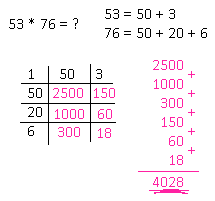## Equations for outputting patterned numbers

Here's a list of how to generate numbers all containing only one digit, two digits, etc. in some pattern. In this case, the variables a,b,c… are single digits that form a number. For example, the number 158 will correspond to a = 1, b = 5, and c = 8. The number 292 corresponds to a = 2 and b = 9, making aba.

```            ```
11 * a = aa
111 * a = aaa
101 * ab = abab
10101 * ab = ababab
111 * a00b = aaabbb
1111 * a000b = aaaabbbb
1001 * abc = abcabc
1001001 * abc = abcabcabc
10001 * abcd = abcdabcd
1111 * a000b000c = aaaabbbbcccc
etc...

```
```

## Strange Patterned Equations

I don't remember how I found these ones (they're in my notes from several years back).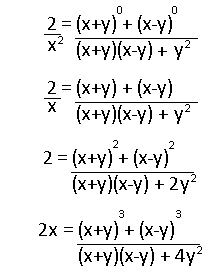Neat little pattern, right? Too bad it stops after the fourth one, unless you consider the following also matching the pattern:It only gets worse from there

## Probability with Divisibility Problem

From a problem a long time ago: Determine the probability that after n spins (n > 1) the product of all the n numbers generated will be divisible by 10. A spin can return the numbers 1 through 9 and all have an equal chance of being picked.

To start off, any combination of numbers multiplied together will be divisible by 10 if it contains a 5 and an even number. So, all we need to worry about is if the spin eventually picks a 5 and some even number at some point. At 1 spin, there is 0 chance of getting a number divisible by 10, then.

At two spins, we're going to branch out all the possibilities for when the first number is picked. So, assume a spin has already happened and one left is needed.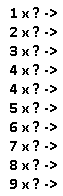For the cases 1, 3, 7, 9, it is already impossible to get a number divisible by 10 with the one remaining spin, so that's a 0 chance there. For the cases 2, 4, 6, 8, it is required to get a 5 on the next spin, which means a (1/9) chance. For the 5 case it is required to get an even number on the next spin, which means a (4/9) chance. Sum up all the probabilities, then divide the result by 9 to account for branching off into 9 equal likely possibilities, and you get the result!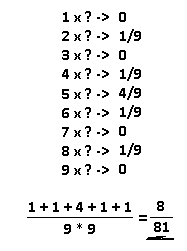What about for three rolls? Well, for the cases 1, 3, 7, 9, you actually end up with the same scenario as with the two spins case, since those numbers contribute nothing to the likelyhood of getting a number divisible by 10. So, the probability is exactly the probability of our previous answer, (8/81). For the cases 2, 4, 6, 8, you just need a 5 to make it. It's easier to calculate the chances of not getting a 5 here, which would be (8/9)*(8/9) = (64/81), then do 1 - (64/81) to get the chances of getting a 5, which is (17/81). For the 5 case, it's the chances of getting an even number in two rolls, and using the same trick in the previous case it's 1 - (5/9)*(5/9) = 56/81.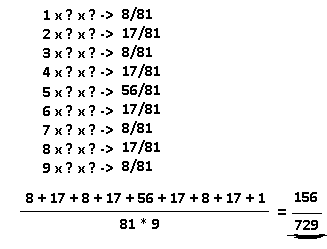Some patterns start showing themselves here. In the cases 1, 3, 7, 9, those probabilities are equal to probability with one less spin. In the cases 2, 4, 6, 8, the chances are always going to be 1 - (8/9)^(N-1), where N is the number of spins. In the 5 case, the chances are always going to be 1 - (5/9)^(N-1). Putting it all together we get a recurrence relation for the probability of getting a number divisible by 10 for N spins.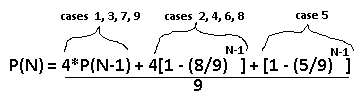Good luck trying to convert it into something that's not a recurrence relation. I'm not sure if it's possible. The function converges to 1 as N grows, which is the main thing that's important, as it should be easier to get a number divisible by 10 as more spins are allowed.